Skip to content →

# Tag: blogging

In the wake of a colleague’s suicide and the suicide of three students, Matilde Marcolli gave an interesting and courageous talk at Caltech in April : The dark heart of our brightness: bipolar disorder and scientific creativity. Although these slides give a pretty good picture of the talk, if you can please take the time to watch it (the talk starts 44 minutes into the video).

Courageous because as the talk progresses, she gives more and more examples from her own experiences, thereby breaking the taboo surrounding the topic of bipolar mood disorder among scientists. Interesting because she raises a couple of valid points, well worth repeating.

We didn’t can see it coming

We are always baffled when someone we know commits suicide, especially if that person is extremely successful in his/her work. ‘(S)he was so full of activity!’, ‘We did not see it coming!’ etc. etc.

Matilde argues that if a person suffers from bipolar mood disorder (from mild forms to full-blown manic-depression), a condition quite common among scientists and certainly mathematicians, we can see it coming, if we look for the proper signals!

We, active scientists, are pretty good at hiding a down-period. We have collected an arsenal of tricks not to send off signals when we feel depressed, simply because it’s not considered cool behavior. On the other hand, in our manic phases, we are quite transparent because we like to show off our activity and creativity!

Matilde tells us to watch out for people behaving orders-of-magnitude out of their normal-mode behavior. Say, someone who normally posts one or two papers a year on the arXiv, suddenly posting 5 papers in one month. Or, someone going rarely to a conference, now spending a summer flying from one conference to the next. Or, someone not blogging for months, suddenly flooding you with new posts…

As scientists we are good at spotting such order-of-magnitude-out-behavior. So we can detect friends and colleagues going through a manic-phase and hence should always take such a person serious (and try to offer help) when they send out signals of distress.

Mood disorder, a Faustian bargain

The Faust legend :
“Despite his scholarly eminence, Faust is bored and disappointed. He decides to call on the Devil for further knowledge and magic powers with which to indulge all the pleasures of the world. In response, the Devil’s representative Mephistopheles appears. He makes a bargain with Faust: Mephistopheles will serve Faust with his magic powers for a term of years, but at the end of the term, the Devil will claim Faust’s soul and Faust will be eternally damned.”

Mathematicians suffering from mood disorder seldom see their condition as a menace, but rather as an advantage. They know they do their best and most creative work in short spells of intense activity during their manic phase and take the down-phase merely as a side effect. We fear that if we seek treatment, we may as well loose our creativity.

That is, like Faust, we indulge the pleasures of our magic powers during a manic-phase, knowing only too well that the devilish depression-phase may one day claim our life or mental sanity…

Via Tanya Khovanova I learned yesterday of the 50 best math blogs for math-majors list by OnlineDegree.net. Tanya’s blog got in 2nd (congrats!) and most of the blogs I sort of follow made it to the list : the n-category cafe (5), not even wrong (6), Gowers (12), Tao (13), good math bad math (14), rigorous trivialities (18), the secret blogging seminar (20), arcadian functor (28) (btw. Kea’s new blog is now at arcadian pseudofunctor), etc., etc. . Sincere congrats to you all!

NeverEndingBooks didn’t make it to the list, and I can live with that. For reasons only relevant to myself, posting has slowed down over the last year and the most recent post dates back from february!

More puzzling to me was the fact that F-un mathematics got in place 26! OnlineDegree had this to say about F-un Math : “Any students studying math must bookmark this blog, which provides readers with a broad selection of undergraduate and graduate concerns, quotes, research, webcasts, and much, much more.” Well, personally I wouldn’t bother to bookmark this site as prospects for upcoming posts are virtually inexistent…

As I am privy to both sites’ admin-pages, let me explain my confusion by comparing their monthly hits. Here’s the full F-un historyAfter a flurry of activity in the fall of 2008, both posting and attendance rates dropped, and presently the site gets roughly 50 hits-a-day. Compare this to the (partial) NeB history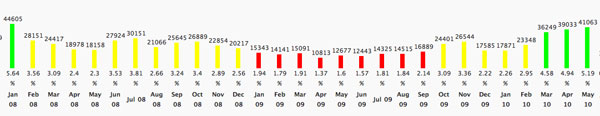The whopping 45000 visits in january 2008 were (i think) deserved at the time as there was then a new post almost every other day. On the other hand, the green bars to the right are a mystery to me. It appears one is rewarded for not posting at all…

The only explanation I can offer is that perhaps more and more people are recovering from the late 2008-depression and do again enjoy reading blog-posts. Google then helps blogs having a larger archive (500 NeB-posts compared to about 20 genuine Fun-posts) to attract a larger audience, even though the blog is dormant.

But this still doesn’t explain why FunMath made it to the top 50-list and NeB did not. Perhaps the fault is entirely mine and a consequence of a bad choice of blog-title. ‘NeverEndingBooks’ does not ring like a math-blog, does it?

Still, I’m not going to change the title into something more math-related. NeverEndingBooks will be around for some time (unless my hard-disk breaks down). On the other hand, I plan to start something entirely new and learn from the mistakes I made over the past 6 years. Regulars of this blog will have a pretty good idea of the intended launch date, not?

Until then, my online activity will be limited to tweets.

To most mathematicians, a good LaTeX-frontend (such as TeXShop for Mac-users) is the crucial tool to get the work done. We use it to draft ideas, write papers and courses, or even to take notes during lectures.

However, after six years of blogging, my own LaTeX-routine became rusty. I rarely open a new tex-document, and when I do, I’d rather copy-paste the long preamble from an old file than to start from scratch with a minimal list of packages and definitions needed for the job at hand. The few times I put a paper on the arXiv, the resulting text resembles a blog-post more than a mathematical paper, here’s an example.

As I desperately need to get some math-writing done, I need to pull myself away from the lure of an ever-open WordPress admin browser-screen and reacquaint myself with the far more efficient LaTeX-environment.

Perhaps even my blogging will benefit from the change. Whereas I used to keep on adding to most of my tex-files in order to keep them up-to-date, I rarely edit a blog-post after hitting the ’publish’ button. If I really want to turn some of my better posts into a book, I need them in a format suitable for neverending polishing, without annoying the many RSS-feed aggregators out there.

Who better than Terry Tao to teach me a more proficient way of blogging? A few days ago, Terry announced he will soon have his 5th (!!) book out, after three years of blogging…

How does he manage to do this? Well, as far as I know, Terry blogs in LaTeX and then uses a python-script called LaTeX2WP ’a program that converts a LaTeX file into something that is ready to be cut and pasted into WordPress. This way, you can write, and preview, your post in LaTeX, then run LaTeX2WP, and post into WordPress whatever comes out.’ More importantly, one retains a pure-tex-file of the post on which one can keep on editing to get it into a (book)-publishable form, eventually.

Nice, but one can do even better, as Eric from Curious Reasoning worked out. He suggests to install two useful python-packages : WordPressLib “with this library you can control remotely a WordPress installation. Use of library is very simple, you can write a small scripts or full applications that allows you to automate publishing of articles on your blog/site powered by WordPress” and plasTeX “plasTeX is a LaTeX document processing framework written entirely in Python. It currently comes bundled with an XHTML renderer (including multiple themes), as well as a way to simply dump the document to a generic form of XML”. Installation is easy : download and extract the files somewhere, go there and issue a **sudo python setup.py install** to add the packages to your python.

Finally, get Eric’s own wplatex package and install it as explained there. WpLaTeX has all the features of LaTeX2WP and much more : one can add titles, tags and categories automatically and publish the post from the command-line without ever having to enter the taboo WordPress-admin page! Here’s what I’ve written by now in TeXShopI’ve added the screenshot and the script will know where to find it online for the blog-version as well as on my hard-disk for the tex-version. Very handy is the iftex … fi versus ifblog … fi alternative which allows you to add pure HTML to get the desired effect, when needed. Remains only to go into Terminal and issue the command

wplpost -x http://www.neverendingbooks.org/xmlrpc.php ReturnToLatex.tex

(if your blog is on WordPress.com it even suffices to give its name, rather than this work-around for stand-alone wordpress blogs). The script asks for my username and password and will convert the tex-file and post it automatic.

To mark the end of 2009 and 6 years of blogging, two musical compositions with a mathematical touch to them. I wish you all a better 2010!

Remember from last time that we identified Olivier Messiaen as the ‘Monsieur Modulo’ playing the musical organ at the Bourbaki wedding. This was based on the fact that his “modes à transposition limitée” are really about epimorphisms between modulo rings Z/12Z→Z/3Z and Z/12Z→Z/4Z.

However, Messiaen had more serious mathematical tricks up his sleeve. In two of his compositions he did discover (or at least used) one of the smaller sporadic groups, the Mathieu group $M_{12}$ of order 95040 on which we have based a whole series of Mathieu games two and a half years ago.

Messiaen’s ‘Ile de fey 2’ composition for piano (part of Quatre études de rythme (“Four studies in rhythm”), piano (1949–50)) is based on two concurrent permutations. The first is shown below, with the underlying motive rotational permutation shown.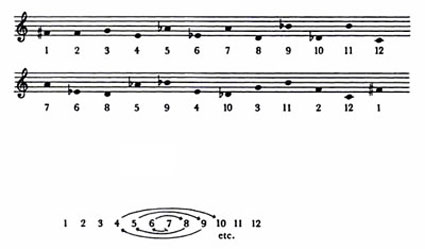This gives the permutation (1,7,10,2,6,4,5,9,11,12)(3,8). A second concurrent permutation is based on the permutation (1,6,9,2,7,3,5,4,8,10,11) and both of them generate the Mathieu group $M_{12}$. This can be seen by realizing the two permutations as the rotational permutations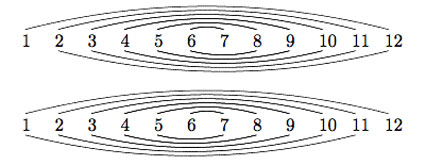and identifying them with the Mongean shuffles generating $M_{12}$. See for example, Dave Benson’s book “Music: A Mathematical Offering”, freely available online.

Clearly, Messiaen doesn’t use all of its 95040 permutations in his piece! Here’s how it sounds. The piece starts 2 minutes into the clip.

The second piece is “Les Yeux dans les Roues” (The Eyes in the Wheels), sixth piece from the “Livre d’Orgue” (1950/51).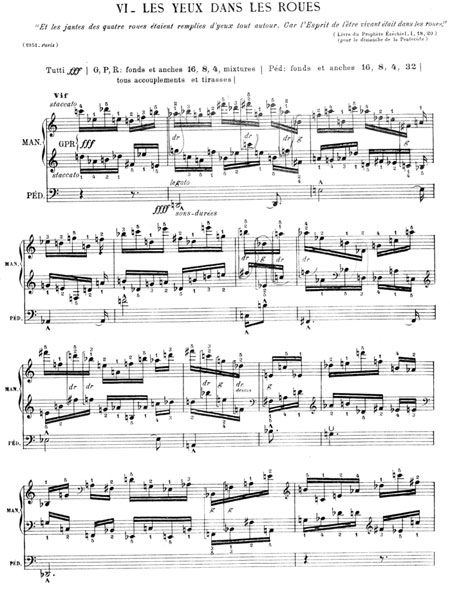According to Hauptwerk, the piece consists of a melody/theme in the pedal, accompanied by two fast-paced homorhythmic lines in the manuals. The pedal presents a sons-durées theme which is repeated six times, in different permutations. Initially it is presented in its natural form. Afterwards, it is presented alternatively picking notes from each end of the original form. Similar transformations are applied each time until the sixth, which is the retrograde of the first. The entire twelve-tone analysis (pitch only, not rhythm) of the pedal is shown below: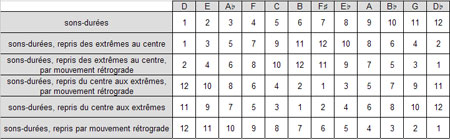That is we get the following five permutations which again generate Mathieu 12 :

• a=(2,3,5,9,8,10,6,11,4,7,12)
• b=(1,2,4,8,9,7,11,3,6,12)(5,10)=e*a
• c=(1,12,11,9,5,4,6,2,10,7)(3,8)=e*d
• d=(1,11,10,8,4,5,3,7,2,9,6)
• e=(1,12)(2,11)(3,10)(4,9)(5,8)(6,7)

Here’s the piece performed on organ :

Considering the permutations $X=d.a^{-1}$ and $Y=(a.d^2.a.d^3)^{-1}$ one obtains canonical generators of $M_{12}$, that is, generators satisfying the defining equations of this sporadic group

$X^2=Y^3=(XY)^{11}=[X,Y]^6=(XYXYXY^{-1})^6=1$

I leave you to work out the corresponding dessin d’enfant tonight after a couple of glasses of champagne! It sure has a nice form. Once again, a better 2010!

A comment-thread well worth following while on vacation was Algebraic Geometry without Prime Ideals at the Secret Blogging Seminar. Peter Woit became lyric about it :

My nomination for the all-time highest quality discussion ever held in a blog comment section goes to the comments on this posting at Secret Blogging Seminar, where several of the best (relatively)-young algebraic geometers in the business discuss the foundations of the subject and how it should be taught.

I follow far too few comment-sections to make such a definite statement, but found the contributions by James Borger and David Ben-Zvi of exceptional high quality. They made a case for using Grothendieck’s ‘functor of points’ approach in teaching algebraic geometry instead of the ‘usual’ approach via prime spectra and their structure sheaves.

The text below was written on december 15th of last year, but never posted. As far as I recall it was meant to be part two of the ‘Brave New Geometries’-series starting with the Mumford’s treasure map post. Anyway, it may perhaps serve someone unfamiliar with Grothendieck’s functorial approach to make the first few timid steps in that directions.Allyn Jackson’s beautiful account of Grothendieck’s life “Comme Appele du Neant, part II” (the first part of the paper can be found here) contains this gem :

“One striking characteristic of Grothendieck’s
mode of thinking is that it seemed to rely so little
on examples. This can be seen in the legend of the
so-called “Grothendieck prime”.

In a mathematical
conversation, someone suggested to Grothendieck
that they should consider a particular prime number.
“You mean an actual number?” Grothendieck
asked. The other person replied, yes, an actual
prime number. Grothendieck suggested, “All right,
take 57.”

But Grothendieck must have known that 57 is not
prime, right? Absolutely not, said David Mumford
of Brown University. “He doesn’t think concretely.””

We have seen before how Mumford’s doodles allow us to depict all ‘points’ of the affine scheme $\mathbf{spec}(\mathbb{Z}[x])$, that is, all prime ideals of the integral polynomial ring $\mathbb{Z}[x]$.
Perhaps not too surprising, in view of the above story, Alexander Grothendieck pushed the view that one should consider all ideals, rather than just the primes. He achieved this by associating the ‘functor of points’ to an affine scheme.

Consider an arbitrary affine integral scheme $X$ with coordinate ring $\mathbb{Z}[X] = \mathbb{Z}[t_1,\ldots,t_n]/(f_1,\ldots,f_k)$, then any ringmorphism
$\phi~:~\mathbb{Z}[t_1,\ldots,t_n]/(f_1,\ldots,f_k) \rightarrow R$
is determined by an n-tuple of elements $~(r_1,\ldots,r_n) = (\phi(t_1),\ldots,\phi(t_n))$ from $R$ which must satisfy the polynomial relations $f_i(r_1,\ldots,r_n)=0$. Thus, Grothendieck argued, one can consider $~(r_1,\ldots,r_n)$ an an ‘$R$-point’ of $X$ and all such tuples form a set $h_X(R)$ called the set of $R$-points of $X$. But then we have a functor

$h_X~:~\mathbf{commutative rings} \rightarrow \mathbf{sets} \qquad R \mapsto h_X(R)=Rings(\mathbb{Z}[t_1,\ldots,t_n]/(f_1,\ldots,f_k),R)$

So, what is this mysterious functor in the special case of interest to us, that is when $X = \mathbf{spec}(\mathbb{Z}[x])$?
Well, in that case there are no relations to be satisfied so any ringmorphism $\mathbb{Z}[x] \rightarrow R$ is fully determined by the image of $x$ which can be any element $r \in R$. That is, $Ring(\mathbb{Z}[x],R) = R$ and therefore Grothendieck’s functor of points
$h_{\mathbf{spec}(\mathbb{Z}[x]}$ is nothing but the forgetful functor.

But, surely the forgetful functor cannot give us interesting extra information on Mumford’s drawing?
Well, have a look at the slightly extended drawing below :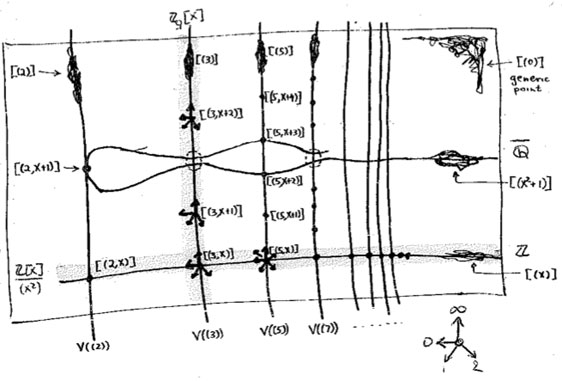What are these ‘smudgy’ lines and ‘spiky’ points? Well, before we come to those let us consider the easier case of identifying the $R$-points in case $R$ is a domain. Then, for any $r \in R$, the inverse image of the zero prime ideal of $R$ under the ringmap $\phi_r~:~\mathbb{Z}[x] \rightarrow R$ must be a prime ideal of $\mathbb{Z}[x]$, that is, something visible in Mumford’s drawing. Let’s consider a few easy cases :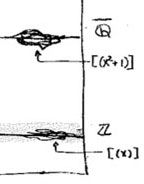For starters, what are the $\mathbb{Z}$-points of $\mathbf{spec}(\mathbb{Z}[x])$? Any natural number $n \in \mathbb{Z}$ determines the surjective ringmorphism $\phi_n~:~\mathbb{Z}[x] \rightarrow \mathbb{Z}$ identifying $\mathbb{Z}$ with the quotient $\mathbb{Z}[x]/(x-n)$, identifying the ‘arithmetic line’ $\mathbf{spec}(\mathbb{Z}) = { (2),(3),(5),\ldots,(p),\ldots, (0) }$ with the horizontal line in $\mathbf{spec}(\mathbb{Z}[x])$ corresponding to the principal ideal $~(x-n)$ (such as the indicated line $~(x)$).

When $\mathbb{Q}$ are the rational numbers, then $\lambda = \frac{m}{n}$ with $m,n$ coprime integers, in which case we have $\phi_{\lambda}^{-1}(0) = (nx-m)$, hence we get again an horizontal line in $\mathbf{spec}(\mathbb{Z}[x])$. For $\overline{\mathbb{Q}}$, the algebraic closure of $\mathbb{Q}$ we have for any $\lambda$ that $\phi_{\lambda}^{-1}(0) = (f(x))$ where $f(x)$ is a minimal integral polynomial for which $\lambda$ is a root.
But what happens when $K = \mathbb{C}$ and $\lambda$ is a trancendental number? Well, in that case the ringmorphism $\phi_{\lambda}~:~\mathbb{Z}[x] \rightarrow \mathbb{C}$ is injective and therefore $\phi_{\lambda}^{-1}(0) = (0)$ so we get the whole arithmetic plane!

In the case of a finite field $\mathbb{F}_{p^n}$ we have seen that there are ‘fat’ points in the arithmetic plane, corresponding to maximal ideals $~(p,f(x))$ (with $f(x)$ a polynomial of degree $n$ which remains irreducible over $\mathbb{F}_p$), having $\mathbb{F}_{p^n}$ as their residue field. But these are not the only $\mathbb{F}_{p^n}$-points. For, take any element $\lambda \in \mathbb{F}_{p^n}$, then the map $\phi_{\lambda}$ takes $\mathbb{Z}[x]$ to the subfield of $\mathbb{F}_{p^n}$ generated by $\lambda$. That is, the $\mathbb{F}_{p^n}$-points of $\mathbf{spec}(\mathbb{Z}[x])$ consists of all fat points with residue field $\mathbb{F}_{p^n}$, together with slightly slimmer points having as their residue field $\mathbb{F}_{p^m}$ where $m$ is a divisor of $n$. In all, there are precisely $p^n$ (that is, the number of elements of $\mathbb{F}_{p^n}$) such points, as could be expected.

Things become quickly more interesting when we consider $R$-points for rings containing nilpotent elements.